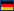In Delphi methods of a class can be called without creating an instance of the class at first. That's similar to C++ static methods. But in C++ it is not possible to assign classes as values to variables and then to create instances of the class by calling a virtual constructor function from such a class reference.

For C++Builder the __classid function is a special extension, to get class references. A small Delphi unit has to be added to the C++Builder project, to create class instances from such a class reference.

To do the same with other compilers is much more difficult.

As for C++Builder where a class TMetaClass is defined, for other compilers such a class is defined too. In addition the type TClass is defined as a pointer to TMetaClass:

```typedef TMetaClass* TClass
```

TMetaClass is the class reference type for TObject and it is the base class of all class reference types of all other classes. These class references are defined as instances of a class ClassRef, which is a generic class:

template <typename Class>
class ClassRef

where the template parameter denotes the original class. That way for a hierarchy of classes, which are derived one from another, there is a parallel hierarchy of class references. The class references are implemented as singletons and only created, if needed. The exact definition of the ClassRef class is tricky and works only, because DelphiXE2Cpp11 also inserts some additional helper code into every class declaration. The following code demonstrates how a small class factory using class references is converted from Delphi to C++:

```type
TBase = class
public
function GetName: String; virtual;
end;
```

``` TBaseClass = class of TBase;
```

``` TDerived = class(TBase)
public
function GetName: String; override;
end;
```

``` TDerivedClass = class of TDerived;
```

```implementation
```

```function make(Base: TBaseClass): TBase;
begin
result := Base.Create;
end;
```

```function testTactory: boolean;
var
s : String;
p : TBase;
begin
p := make(TDerived1);
result := p.GetName = 'TDerived1';
end;
```

##### ->

```class TBase : public System::TObject
{                                                                                                                            public:
typedef System::ClassRef ClassRefType;
ClassRefType* ClassType() const {return  System::class_id();}
static TBase* Create() {return new TBase();}
static System::String ClassName() {return L"TBase";}
virtual System::String GetName();
TBase();
};
```

```typedef TBase::ClassRefType TBaseClass;
```

```class TDerived : public TBase
{
public:
typedef System::ClassRef ClassRefType;
ClassRefType* ClassType() const {return  System::class_id();}
static TDerived* Create() {return new TDerived();}
static System::String ClassName() {return L"TDerived";}
virtual System::String GetName();
TDerived1();
};
```

```typedef TDerived::ClassRefType TDerivedClass;
```

```TBase* make(TBaseClass* Base)
{
TBase* result = nullptr;
result = Base->Create();
return result;
}
```

```bool testfactory()
{
bool result = false;
String s;
TBase* P = nullptr;
P = make(class_id());
result = P->GetName() == L"TDerived";
return result;
}
```

The central point in this code is the call of the class_id-function:

```P = make(class_id());
```

The class_id-function fulfills the same purpose as the __classid-function in C++Builder code: it delivers class reference. In the example the class_id-function delivers the class reference to the class TDerived.Deutsch

 Latest News 11/15/19 DelphiXE2Cpp11 nifty class references offer [more...] 10/16/19 Delphi2C# beta offer [more...]